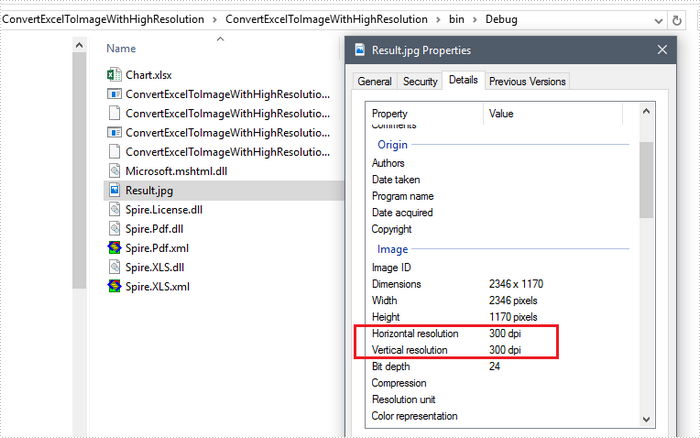# Convert Excel Sheet to a High-Resolution Image in C#, VB.NET

Sometimes, you may want to convert Excel sheet to a high-resolution image, especially when the Excel report contains graphs or pictures. This article will show you how to set image resolution when saving Excel sheet to JPG using Spire.XLS.

Step 1: Create a custom function that you can use to reset the image resolution.

```private static Bitmap ResetResolution(Metafile mf, float resolution)
{
int width = (int)(mf.Width * resolution / mf.HorizontalResolution);
int height = (int)(mf.Height * resolution / mf.VerticalResolution);
Bitmap bmp = new Bitmap(width, height);
bmp.SetResolution(resolution, resolution);
Graphics g = Graphics.FromImage(bmp);
g.DrawImage(mf, 0, 0);
g.Dispose();
return bmp;
}
```

Step 2: Create a workbook instance and load the sample Excel file.

```Workbook workbook = new Workbook();
```

Step 3: Get the worksheet you want to convert.

```Worksheet worksheet = workbook.Worksheets;
```

Step 4: Convert the worksheet to EMF stream.

```MemoryStream ms = new MemoryStream();
worksheet.ToEMFStream(ms, 1, 1, worksheet.LastRow, worksheet.LastColumn);
```

Step 5: Create an image from the EMF stream, and call ResetResolution to reset the resolution for the image.

```Image image = Image.FromStream(ms);
Bitmap images = ResetResolution(image as Metafile, 300);
```

Step 6: Save the image in JPG file format.

```images.Save("Result.jpg", ImageFormat.Jpeg);
```

Output:Full Code:

[C#]
```using Spire.Xls;
using System.Drawing;
using System.Drawing.Imaging;
using System.IO;
namespace Convert
{
class Program
{
static void Main(string[] args)
{
Workbook workbook = new Workbook();
Worksheet worksheet = workbook.Worksheets;

using (MemoryStream ms = new MemoryStream())
{

worksheet.ToEMFStream(ms, 1, 1, worksheet.LastRow, worksheet.LastColumn);
Image image = Image.FromStream(ms);
Bitmap images = ResetResolution(image as Metafile, 300);
images.Save("Result.jpg", ImageFormat.Jpeg);
}
}
private static Bitmap ResetResolution(Metafile mf, float resolution)
{
int width = (int)(mf.Width * resolution / mf.HorizontalResolution);
int height = (int)(mf.Height * resolution / mf.VerticalResolution);
Bitmap bmp = new Bitmap(width, height);
bmp.SetResolution(resolution, resolution);
Graphics g = Graphics.FromImage(bmp);
g.DrawImage(mf, 0, 0);
g.Dispose();
return bmp;
}
}
}
```
[VB.NET]
```Imports Spire.Xls
Imports System.Drawing
Imports System.Drawing.Imaging
Imports System.IO
Namespace Convert
Class Program
Private Shared Sub Main(args As String())
Dim workbook As New Workbook()
Dim worksheet As Worksheet = workbook.Worksheets(0)

Using ms As New MemoryStream()

worksheet.ToEMFStream(ms, 1, 1, worksheet.LastRow, worksheet.LastColumn)
Dim image__1 As Image = Image.FromStream(ms)
Dim images As Bitmap = ResetResolution(TryCast(image__1, Metafile), 300)
images.Save("Result.jpg", ImageFormat.Jpeg)
End Using
End Sub
Private Shared Function ResetResolution(mf As Metafile, resolution As Single) As Bitmap
Dim width As Integer = CInt(mf.Width * resolution / mf.HorizontalResolution)
Dim height As Integer = CInt(mf.Height * resolution / mf.VerticalResolution)
Dim bmp As New Bitmap(width, height)
bmp.SetResolution(resolution, resolution)
Dim g As Graphics = Graphics.FromImage(bmp)
g.DrawImage(mf, 0, 0)
g.Dispose()
Return bmp
End Function
End Class
End Namespace
```